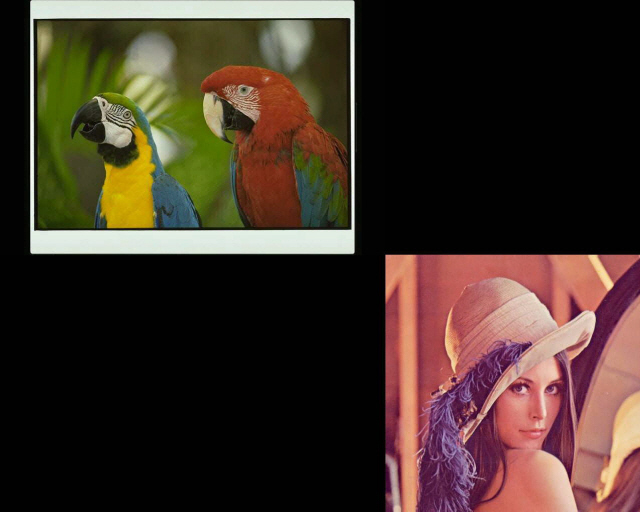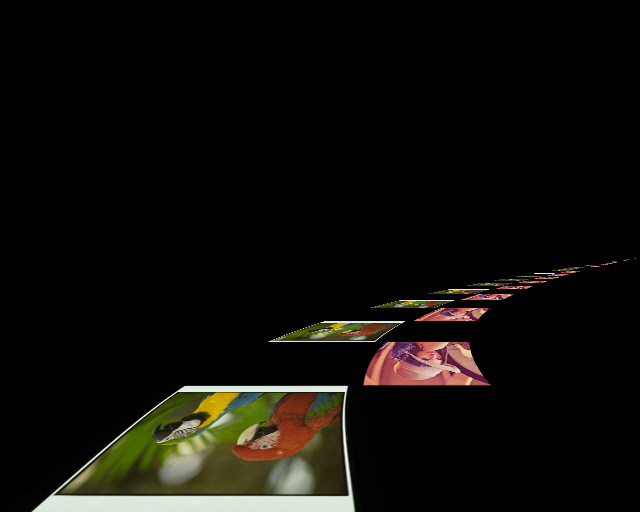﻿ PlaneBendCommand Class | Leadtools.ImageProcessing.SpecialEffects | Raster, Medical, Document Help
←Select platform
In This Topic ▼

# PlaneBendCommand Class

Summary

Places the image on parallel planes along the Z-axis, and bends the planes toward the center point. This command is available in the Imaging Pro and above toolkits.

Syntax
C#
VB
C++
``public class PlaneBendCommand : RasterCommand ``
````  `
`Public Class PlaneBendCommand  `
`   Inherits RasterCommand ````
``public ref class PlaneBendCommand : public RasterCommand   ``
Remarks
• This command shows the effect of placing images on planes along the Z-axis and bending them toward a specific point. These images may be placed above, below, to the left or to the right of the Z-axis. An external light source of the specified color and brightness may be shined on the images. The brightness may vary along the z-axis according to the bright length value. The line of images may be bent toward a specific point by setting a bending value.
• By changing the center point and the viewing screen Z-offset, you can simulate moving along the axis.
• If the image has a region, the effect will be applied on the region dimensions only.
• For an example, see the following figure:The following figure shows the same image, after the effect has been applied:To obtain this effect, the following settings were used with the method:

2. ZValue = 0
3. Distance = 256
4. PlaneOffset = 256
5. Repeat = -1
6. PyramidAngle = 0
7. Stretch = 100
8. BendFactor = 500
9. StartBright = 0
10. EndBright = 0
11. BrightLength = 50
12. BrightColor = new Leadtools.RasterColor(255, 255, 255)
13. FillColor = new Leadtools.RasterColor(0, 0, 0)
14. Flags = PlaneCommandFlags.Down | PlaneCommandFlags.Color
15. This command does not support 32-bit grayscale images.
• This command supports 12-bit and 16-bit grayscale and 48-bit and 64-bit color images. Support for 12-bit and 16-bit grayscale and 48-bit and 64-bit color images is available only in the Document/Medical toolkits.

• This command does not support signed data images.

Example

Run the PlaneBendCommand on an image.

C#
VB
````using Leadtools; `
`using Leadtools.Codecs; `
`using Leadtools.ImageProcessing.SpecialEffects; `
` `
`public void PlaneBendCommandExample() `
`{ `
`   // Load an image `
`   RasterCodecs codecs = new RasterCodecs(); `
`   codecs.ThrowExceptionsOnInvalidImages = true; `
` `
`   RasterImage image = codecs.Load(Path.Combine(LEAD_VARS.ImagesDir, "sample5.cmp")); `
` `
`   // Prepare the command `
`   PlaneBendCommand command = new PlaneBendCommand(); `
`   command.CenterPoint = new LeadPoint(image.Width / 2, image.Height / 2); `
`   command.ZValue = 0; `
`   command.Distance = image.Height; `
`   command.PlaneOffset = image.Width / 2; `
`   command.Repeat = -1; `
`   command.PyramidAngle = 0; `
`   command.Stretch = 100; `
`   command.BendFactor = 400; `
`   command.StartBright = 0; `
`   command.EndBright = 100; `
`   command.BrightLength = 20000; `
`   command.BrightColor = new RasterColor(255, 255, 255); `
`   command.FillColor = new RasterColor(0, 0, 0); `
`   command.Flags = PlaneCommandFlags.Down | PlaneCommandFlags.Up | PlaneCommandFlags.Color; `
`   command.Run(image); `
`   codecs.Save(image, Path.Combine(LEAD_VARS.ImagesDir, "Result.jpg"), RasterImageFormat.Jpeg, 24); `
` `
`} `
` `
`static class LEAD_VARS `
`{ `
`   public const string ImagesDir = @"C:\Users\Public\Documents\LEADTOOLS Images"; `
`} ````
````Imports Leadtools `
`Imports Leadtools.Codecs `
`Imports Leadtools.ImageProcessing.SpecialEffects `
` `
`Public Sub PlaneBendCommandExample() `
`   Dim codecs As New RasterCodecs() `
`   codecs.ThrowExceptionsOnInvalidImages = True `
` `
`   Dim leadImage As RasterImage = codecs.Load(Path.Combine(LEAD_VARS.ImagesDir, "sample5.cmp")) `
` `
`   ' Prepare the command `
`   Dim command As PlaneBendCommand = New PlaneBendCommand `
`   command.CenterPoint = New LeadPoint(leadImage.Width \ 2, leadImage.Height \ 2) `
`   command.ZValue = 0 `
`   command.Distance = leadImage.Height `
`   command.PlaneOffset = leadImage.Width \ 2 `
`   command.Repeat = -1 `
`   command.PyramidAngle = 0 `
`   command.Stretch = 100 `
`   command.BendFactor = 400 `
`   command.StartBright = 0 `
`   command.EndBright = 100 `
`   command.BrightLength = 20000 `
`   command.BrightColor = New RasterColor(255, 255, 255) `
`   command.FillColor = New RasterColor(0, 0, 0) `
`   command.Flags = PlaneCommandFlags.Down Or PlaneCommandFlags.Up Or PlaneCommandFlags.Color `
`   command.Run(leadImage) `
`   codecs.Save(leadImage, Path.Combine(LEAD_VARS.ImagesDir, "Result.jpg"), RasterImageFormat.Jpeg, 24) `
` `
`End Sub `
` `
`Public NotInheritable Class LEAD_VARS `
`   Public Const ImagesDir As String = "C:\Users\Public\Documents\LEADTOOLS Images" `
`End Class ````
Requirements